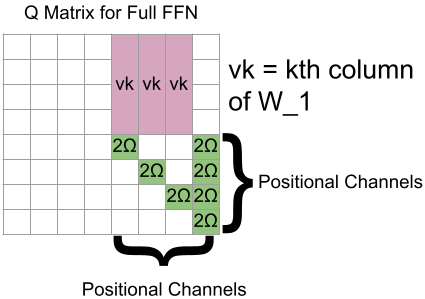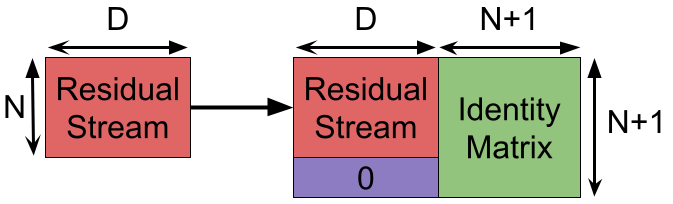# Addendum: More Efficient FFNs via Attention

post by Robert_AIZI · 2023-02-06T18:55:25.893Z · LW · GW · 2 comments

## Contents

  Perspective 1 - Identical Steps
Perspective 2 - Virtual Attention Heads
Summary
Demonstration Code
Conclusion
None


[Epistemic status: I have running code that implements it.]

Overview: I previously showed how an FFN layer in a transformer can be implemented via 3 attention layers. [LW · GW] In this post I show how to do it in a single attention layer. This reduces the needed dimensionality of your model from 5D+N+1 to D+N+1. The main bottleneck, needing 4D attention heads for the hidden layers, remains.

Hot Take: [epistemic status: much less confidence than the rest of this post] The bottleneck - that one needs 4D attention heads for the hidden layers - could be capturing a mechanistic interpretability insight: the FFN components of transformers are less interpretable simply because they consist of ~500x more attention heads than a traditional attention layer. This could suggest a “scale is all you need” approach to mechanistic interpretability - we’ll be able to understand large attention-only models if and only if we can understand smaller FFN+attention models.

Outline: I’ll cover two perspectives that helped me realize you could do this simplification, then summarize the changes, link to the code, then give some concluding thoughts.

I will assume you are familiar with the previous post and it’s notation, so if read it here if you need a refresher.

## Perspective 1 - Identical Steps

I first realized we could simplify this by imagining the perspective of a single entry in the hidden layer of a transformer’s FFN. We:

• (F1) Compute the entry via linear operation on the residual stream
• (F2) Apply a nonlinearity (the activation function)
• (F3) Write to the residual stream via a linear operation

Compare with the steps in an attention head:

• (A1) Compute pre-attention via a linear operation on the residual stream
• (A2) Apply a nonlinearity (the rowwise softmax)
• (A3) Write to the residual stream via a linear operation

Suspiciously similar! In my previous post, I used separate attention layers for F1, F2, and F3, but one can actually choose Q and V matrices so that A1/2/3 computes F1/2/3, respectively, allowing you to complete the FFN in a single attention layer.

## Perspective 2 - Virtual Attention Heads

A Mathematical Framework for Transformer Circuits introduced “virtual attention heads”, which provide another useful intuition.

In short, attention heads in two consecutive layers can (in some sense) be treated as a single combined “virtual” attention head. Writing  for the attention patters and  for the weights being written to the residual stream, attention heads are characterized by , and the virtual attention head produced by  and  is , with the caveat that the attention pattern from layer 1 influences the attention pattern in layer 2.

Since this part is just to build intuition, we’re going to play fast and loose with notation and matrix sizes. But applying this analysis to the linear, SiLU, and linear sublayers described in the previous post, we get:

• : For the first linear layer, we forced every vector to only attend to itself, so we have  (the identity matrix). Our  matrix contained a copy of the weight matrix , so in an abuse of notation let us write .
• : For the SiLU layer, our  matrix is complicated. But our  matrix is a matrix unit , where  is the dimension being SiLU’d.
• : For the second linear layer, we forced every vector to only attend to itself, so we have  (the identity matrix). Our  matrix contained a copy of the weight matrix , so in an abuse of notation let us write .

Now, thinking in terms of virtual attention heads, we have . Since , this simplifies to .

When one does this analysis rigorously, there are three nuances we must add:

1. Since  is size D-by-D, it must be padded out with 0s to make it side D’-by-D’ (here, ). That is , where  means “put matrix  in the upper left corner of a new matrix and add 0s to make it the right-sized square matrix”.
2. Previously we computed  as , resulting in negative signs in  and in . However, in this approach we compute  directly, so those negative signs go away.
3. The  matrix computes attention patterns from the residual stream after it was modified by , so the previous -1 entries are replaced with the th column of the  matrix. (No such accounting has to happen for the  matrix, since we force  to be the identity matrix no matter what.)

## Summary

The resulting Q matrix for computing attention looks like this:The Q matrix to calculate the output of the FFN’s kth hidden dimension.

And as mentioned before, , where  and  are the weight matrices for your FFN as before, and  is the 4D-by-4D matrix with a 1 in the th spot and a 0 elsewhere.

You use one such attention head for each of the 4D hidden dimensions. For GPT-3, that is a crushing 49152 attention heads in the FFN layer, compared to 96 attention heads in a normal attention layer. This a major slowdown compared to computing an FFN normally, although these attention heads could be parallelized.

Since we compute the hidden layers within the attention heads, we no longer need 4D extra dimensions in our model to store those values between steps. Now the model dimension is D+N+1 (the N+1 channels being used for 1-hot positional encoding). For GPT-3, that raises the dimensionality from 12288 to 14337, a 17% increase.The residual stream is augmented from size N-by-D to size (N+1)-by-(D+N+1).

## Demonstration Code

I’ve put Python code implementing this technique on github. Each of the now two components (FFNs, normal attention) are implemented both directly and with attention heads. They are tested on random matrices with  and , and the largest error entries in each matrix are on the order of . I have not tested how such errors propagate through multiple layers.

## Conclusion

(To be read as a supplement to the conclusions in the previous post, which still stand.)

• [Epistemic status: high confidence] It is now somewhat more feasible to use this technique to augment transparency tools on transformers.
• Compared to the previous technique, we have reduced the number of attention layers by a factor of 2x.
• Compared to the previous technique, we have reduced the dimensionality of the residual stream by a factor of ~5x.
• [Epistemic status: high confidence] On GPT-3’s size hyperparameters, this technique would produce ~50k attention heads per FFN sublayer, more than 500x the number of attention heads in GPT-3’s classic attention layer!
• [Epistemic status: high confidence] This gives a different perspective on the balance between attention heads and FFNs in LLMs.
• This is a useful reminder that each hidden dimension in an FFN operates independently of the others, just as a group of attention heads work independently in parallel with each other.
• [epistemic status: much less confidence than the rest of this post] It is possible that previous work “had much less success in understanding MLP layers so far” precisely because of this difference in scale - to study internal attention heads increases the number of attention heads by almost 3 orders of magnitude.
• We could test this hypothesis if we had interpretability tools that work across multiple orders of magnitude. For example, if we had interpretability tools that work on 10000-headed attention-only transformers, we could apply them to 20-headed attention+FFN transformers, and expect roughly similar success in interpretability.
• [Epistemic status: joke] I just had a great, extremely original idea for a slogan for such an interpretability paradigm: “scale is all you need”.